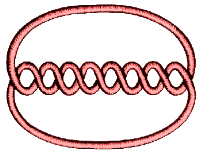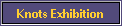# Prime Knots

The analogy between knots and numbers goes deeper.
A prime number is one that is not a product of smaller numbers. The first few prime numbers are

2, 3, 5, 7, 11, 13, 17, 19, 23, 29, 31, 37, . . . .

Any number can be written as a product of a set of prime numbers. Here is an example:

60 = 2 x 2 x 3 x 5 = 2 x 5 x 3 x 2.

The number 60 determines the list 2, 2, 3, 5 of primes, but not the order in which they are used.

The same is true for knots.

A prime knot is one that is not the sum of simpler knots.
Examples of prime knots include:. . . . .

Any knot with bridge number equal to 2 is a prime knot.

The trefoil and the cinquefoil are members of the family that contains the knot:These are the torus knots. All the torus knots are prime knots, so there are lots of prime knots.

Any knot can be written as a sum of a list of prime knots. These prime knots are determined by the original knot, but in practice it can be very difficult to work out what these prime knots will be.

Decomposition into simple elements is a basic process in mathematics, or indeed wherever one deals with complicated matters. In knot theory the process crops up in a variety of guises.

We have already mentioned the prime decomposition of knots. Here the prime knots are the simple elements and the fact that any knot can be expressed uniquely (up to order) as a sum of prime knots is clearly an important fact about knots.

The process of transforming one diagram of a knot into another may be quite complicated. It is therefore of interest that such a complex process can be resolved into a sequence of one or other of four simple changes of crossings, the Reidemeister movestogether with deforming without changing the crossings. We illustrated this in the process of changing the Bowline, and also in illustrating why the colourability of a knot is an invariant.

There are an infinite number of sequences of moves that you can perform on a diagram. That is why it can be difficult to decide if two diagrams do or do not represent the same knot. If they do represent the same knot, it can be difficult to decide the smallest number of moves that can be used to transform one diagram into another.

| Previous | Next |© Mathematics and Knots, U.C.N.W.,Bangor, 1996 - 2002
This material may be used freely for educational, artistic and scientific purposes, but may not be used for commercial purposes, for profit or in texts without the permission of the publishers.